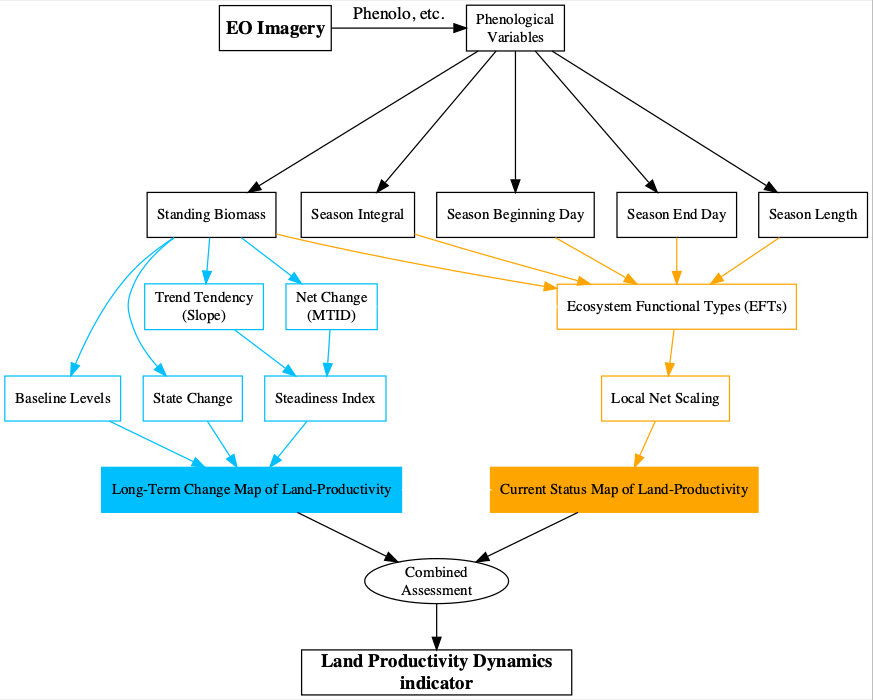# Land Productivity Dynamics: a simple example with LPDynR

## Introduction

As part of the UN Sustainable Development Goal 15 (Life on Land), the indicator 15.3.1 is adopted to measure the Land Degradation Neutrality (stable —or increasing— state regarding the amount and quality of land resources required to support ecosystem functions and services and enhance food security during a certain period of time). It is a binary indicator (i.e. degraded/not degraded), expressed as the proportion (%) of land that is degraded over total land area, and is based on three sub-indicators: (1) Trends in Land Cover, (2) Land Productivity and (3) Carbon Stocks.

The Land Productivity sub-indicator (LP) refers to the total above-ground Net Primary Production and reflects changes in health and productive capacity of the land. Its declining trends can be usually understood as land degradation. LP is calculated using the Land Productivity Dynamics (LPD) approach, first developed by Ivits and Cherlet (2013). The LPD approach uses phenological and productivity variables derived from time series of remote sensed imagery, particularly the normalized difference vegetation index (NDVI), to estimate ecosystem dynamics and change.

LPD is the methodological basis of the LPDynR package. It is based on a combined assessment of two sources of information, as seen in Figure 1. On the one hand, the first layer is the Long Term Change Map and, in general terms, it shows the tendency of change of land productivity (positive or negative) and the effect that this tendency might have had on a particular original point after a certain period of time. On the other hand, the second layer is the Current Status Map, which provides information on the current efficiency levels of vegetation on the productivity or, in other words, the current level of land productivity in relation to its potential. Further explanations for both branches can be found in Rotllan-Puig, et al. (2021). The final result of the indicator is a categorical map with 5 classes of land productivity dynamics, ranging from declining to increasing productivity.Figure1: Flowchart of the process to calculate the Land Productivity Dynamics indicator and followed by LPDynR

In this vignette we show a simple example on how to calculate the LPD indicator using LPDynR.

## Example

library(devtools)
install_github("xavi-rp/LPDynR")
library(LPDynR)
library(terra)

Loading a land productivity variable derived from Earth Observation imagery. A SpatRaster object with time series (each layer is one year).

variables_dir <- "yourDirectoryPath/"   # directory where land productivity and phenological variables are (SpatRaster objects with time series)

sb <- rast(paste0(variables_dir, "/standingBiomass.tif"))    # Standing biomass (integral between the two minimas)

Or, in case you want to use the example data sets included in LPDynR:

variables_dir <- paste0(system.file(package='LPDynR'), "/extdata/")   # directory

?sb_cat
sb <- rast(paste0(system.file(package='LPDynR'), "/extdata/sb_cat.tif")) # Standing biomass (integral between the two minimas)

1. Steadiness Index (trend tendency + net change).
?steadiness

cores2use = 3,  # for parallel processing
filename = "SteadInd.tif")

1. Baseline Level. The user has to define the proportion of drylands over the total land. As examples, in Europe drylands cover 20% of total land (FAO, 2019); globally, 40 percent of the World’s land resources are drylands (Middleton et al., 2011).
?baseline_lev

Baseline_Level <- baseline_lev(obj2process = sb,
yearsBaseline = 3,
drylandProp = 0.4,    # 40% of total land
highprodProp = 0.1,   # 10% of total land
cores2use = 3,
filename = "Baseline_Level.tif")

1. State Change.
?state_change

State_Change <- state_change(obj2process = sb,
yearsBaseline = 3,
cores2use = 3,
filename = "State_Change.tif")

1. Long Term Change Map.
?LongTermChange

BaselineLevels = Baseline_Level,
StateChange = State_Change,
filename = "Long_Term_Change_Map.tif")

1. Renoving multicollinearity among variables.
?rm_multicol

variables_noCor <- rm_multicol(dir2process = variables_dir,
multicol_cutoff = 0.7,
cores2use = 3,
filename = "variables_noCor.tif")

1. PCAs: Preparing variables for clustering.
?PCAs4clust

pca_final_brick <- PCAs4clust(obj2process = variables_noCor,
cumul_var_threshold = 0.9,
filename = "pca_final_brick.tif")

1. Ecosystem Functional Types (EFTs).
?clust_optim
?EFT_clust

# Producing a scree plot with number of cluster at x-axis and total within-cluster sum of squares at y-axis.
# The 'scree plot method' allows the user to assess how the quality of the K-means clustering improves when
# increasing the number of clusters. An elbow in the curve indicates the optimal number of clusters

jpeg("OptimalNumClusters.jpg")
clust_optim(obj2clust = pca_final_brick,
num_clstrs = seq(5, 50, 5))
dev.off()

# Deriving EFTs with the optimal number of clusters calculated before

EFTs <- EFT_clust(obj2clust = pca_final_brick,
n_clust = 12,
nstart = 5,
algorithm = "Hartigan-Wong",
filename = "EFTs.tif")

clust_eval <- EFTs[]     # Evaluation of clustering performance
EFTs <- EFTs[]           # SpatRaster object with the clusters (i.e. EFTs)

1. Local net productivity scaling (LNS). Current Net Primary Production relative to its potential.
?LNScaling

# Productivity variable
si <- rast(paste0(variables_dir, "/cyclicFraction.tif"))    # Season integral (seasonal growth)

LNScal <- LNScaling(EFTs = EFTs,
ProdVar = si,
cores2use = 3,
filename = "LNScal.tif")

1. Land Productivity Dynamics Combined Assessment. The final product, a SpatRaster object.
?LPD_CombAssess

LPD_finalMap <- LPD_CombAssess(LandProd_change = "Long_Term_Change_Map",
LandProd_current =  "LNScal",
filename = "LPD_finalMap.tif")
plot(LPD_finalMap)